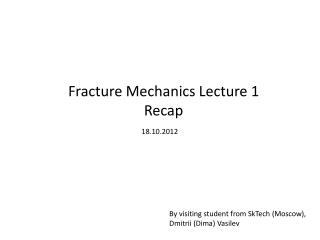DownloadDownload PresentationFracture Mechanics Lecture 1 Recap

# Fracture Mechanics Lecture 1 Recap

Download Presentation## Fracture Mechanics Lecture 1 Recap

- - - - - - - - - - - - - - - - - - - - - - - - - - - E N D - - - - - - - - - - - - - - - - - - - - - - - - - - -
##### Presentation Transcript

1. Fracture Mechanics Lecture 1 Recap 18.10.2012 By visiting student from SkTech (Moscow), Dmitrii (Dima) Vasilev

2. We started with the problem to be solved in fracture mechanics • What is the magnitude of the load that will cause the body (material) to fracture? Let us assume we are creating a new material Then,

3. The procedure to answer the question is the following • What is the maximum stress in the body? • What is the strength of material? • When τ(max) = X(Material Strength) – material fractures

4. To proceed we need to use Theory of Linear Elasticity Let’s assume we found each stress component for each particle Then we find maximum stresses from eigenvalues

5. Stress concentration • It turns out that in some specific areas of the body the stresses may be higher than the applied load F Stress concentration Stress concentration F

6. Due to linearity… • τ(max)=c*τ(app) C=1+2a/b C=3 F Stress concentration Stress concentration F

7. Strength of material • Theory cannot accurately predict strength But • We can conduct experiments

8. However… • The maximum stress in a body is sensitive to the shape of the flaw • The shape of the flaw inside the body is seldom known • We assume linear elasticity • Strength depends on the sample shape

9. Griffith attempts Some experiments were conducted on the glass sample. Conclusions: • In a body of glass cracks pre-exist. • The tip of such crack concentrate stresses. • The intense stress breaks atomic bonds one by one, like opening a zipper. • As the crack advances,

10. The main conclusion is that there is a competition between 2 factors while the crack advances: releasing elastic energy forces the crack to grow, while surface crack energy (which grows) is trying to stop the crack expansion (to heal the crack). U a*=2γE/πσ^2 a

11. Why the discussed material is tremendously important?

12. My previous work 300 tons of liquid steel New material for ladle lining – Carbonized Periclase, which has a thermal expansion coefficient significantly higher than previous materials

13. What I found

14. Video of numerical methods that I used to solve that problem